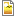# Datapoint interpolation

This is a submodel that calculates an interpolated value for a function defined by a fixed, irregularly spaced set of data points. It does simple linear interpolation, extrapoilating the line between the last two datapoints at either end to cover the domain outside the spread of datapoints. The descriptions are oriented to its use to calculate interpolated values from a time series, but the independent variable called CurrentTime could actually be any argument for the interpolated function.

Include this in your model, and make sure the submodel 'Datapoint' is set to the same number of instances as you have time points in your file.

Load the two fixed parameters with the data from your columns of time points and values respectively. Hint: if you do not drag any headings into the indices table, it will use the row number as the index. The rest of the model segment works out the interpolated value for the current time by finding the indices of the last and next time points and scaling the values at those times.data-interp.png18.6 KB